# Plotting whitened data#

This tutorial demonstrates how to plot whitened evoked data.

Data are whitened for many processes, including dipole fitting, source localization and some decoding algorithms. Viewing whitened data thus gives a different perspective on the data that these algorithms operate on.

Let’s start by loading some data and computing a signal (spatial) covariance that we’ll consider to be noise.

```import mne
from mne.datasets import sample
```

## Raw data with whitening#

Note

In the `mne.io.Raw.plot()` with `noise_cov` supplied, you can press they “w” key to turn whitening on and off.

```data_path = sample.data_path()
raw_fname = data_path / "MEG" / "sample" / "sample_audvis_filt-0-40_raw.fif"

events = mne.find_events(raw, stim_channel="STI 014")
event_id = {
"auditory/left": 1,
"auditory/right": 2,
"visual/left": 3,
"visual/right": 4,
"smiley": 5,
"button": 32,
}
epochs = mne.Epochs(raw, events, event_id=event_id, reject=reject)

# baseline noise cov, not a lot of samples
noise_cov = mne.compute_covariance(
epochs, tmax=0.0, method="shrunk", rank=None, verbose="error"
)

# butterfly mode shows the differences most clearly
raw.plot(events=events, butterfly=True)
raw.plot(noise_cov=noise_cov, events=events, butterfly=True)
```
•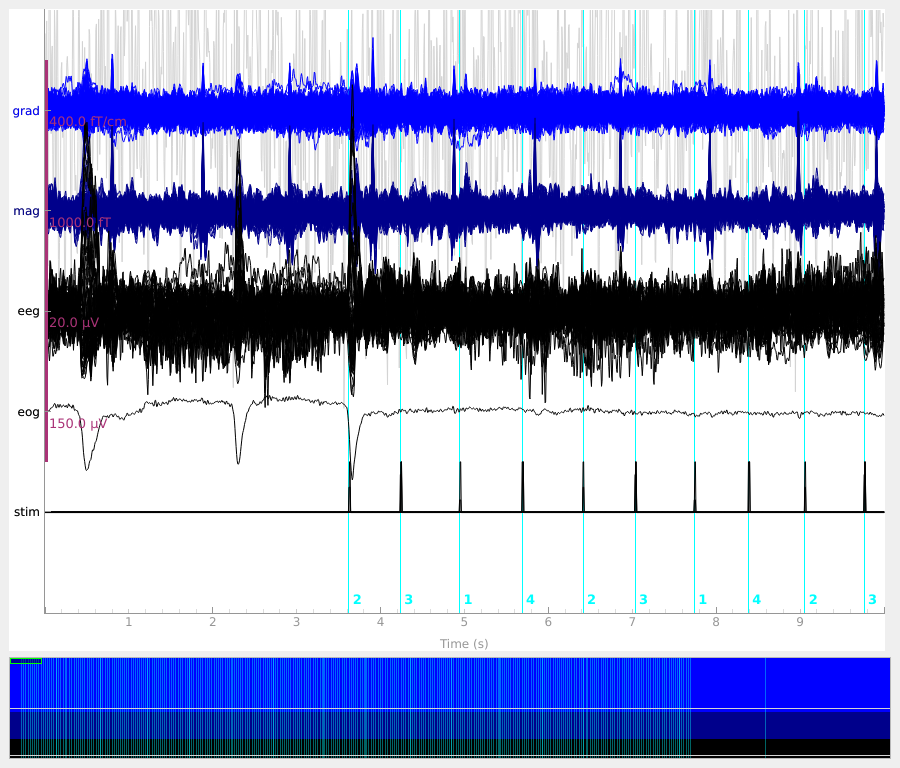•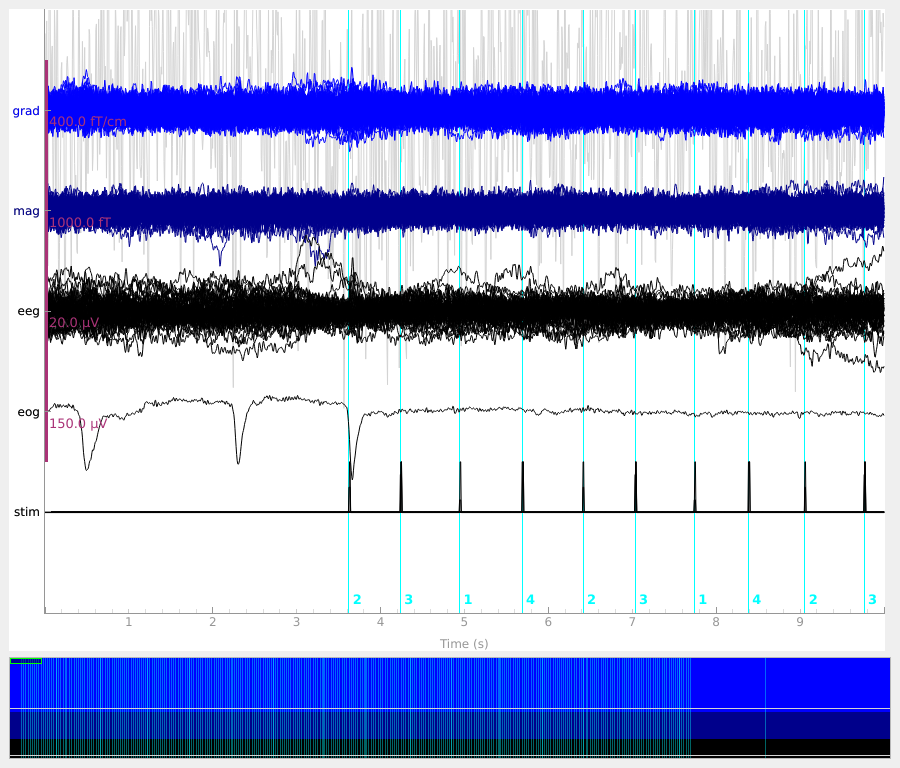```Opening raw data file /home/circleci/mne_data/MNE-sample-data/MEG/sample/sample_audvis_filt-0-40_raw.fif...
Read a total of 4 projection items:
PCA-v1 (1 x 102)  idle
PCA-v2 (1 x 102)  idle
PCA-v3 (1 x 102)  idle
Average EEG reference (1 x 60)  idle
Range : 6450 ... 48149 =     42.956 ...   320.665 secs
Reading 0 ... 41699  =      0.000 ...   277.709 secs...
319 events found
Event IDs: [ 1  2  3  4  5 32]
319 matching events found
Setting baseline interval to [-0.19979521315838786, 0.0] s
Applying baseline correction (mode: mean)
Created an SSP operator (subspace dimension = 4)
4 projection items activated
Computing rank from covariance with rank=None
Using tolerance 8.3e-14 (2.2e-16 eps * 59 dim * 6.3  max singular value)
Estimated rank (eeg): 58
EEG: rank 58 computed from 59 data channels with 1 projector
Computing rank from covariance with rank=None
Using tolerance 1.9e-13 (2.2e-16 eps * 203 dim * 4.3  max singular value)
GRAD: rank 203 computed from 203 data channels with 0 projectors
Computing rank from covariance with rank=None
Using tolerance 2.7e-14 (2.2e-16 eps * 102 dim * 1.2  max singular value)
Estimated rank (mag): 99
MAG: rank 99 computed from 102 data channels with 3 projectors
```

## Epochs with whitening#

```epochs.plot(events=True)
epochs.plot(noise_cov=noise_cov, events=True)
```
•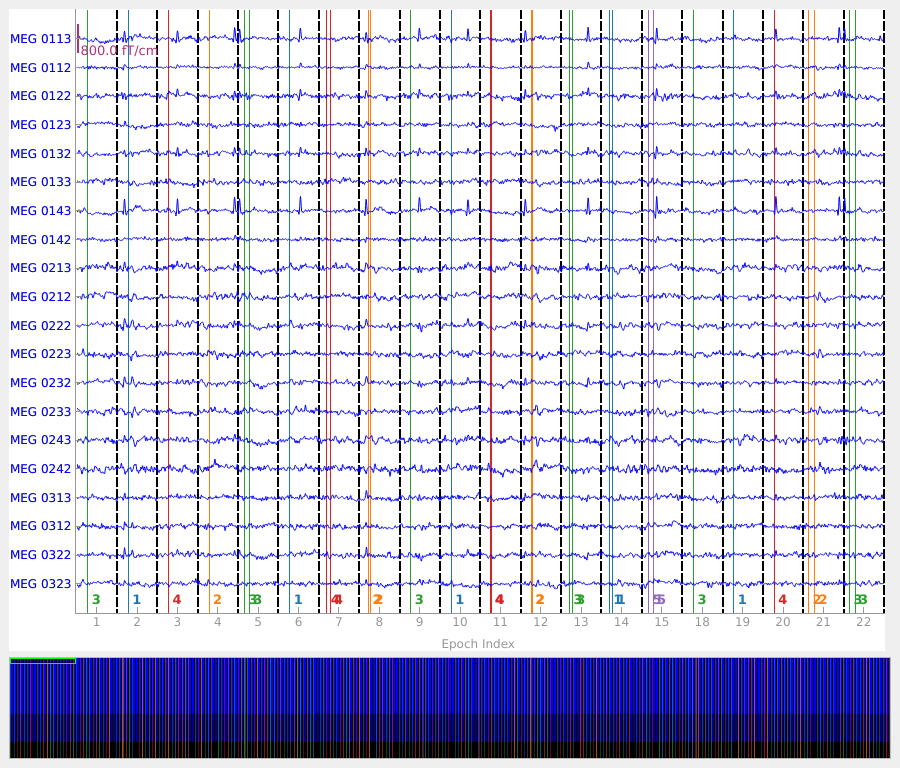•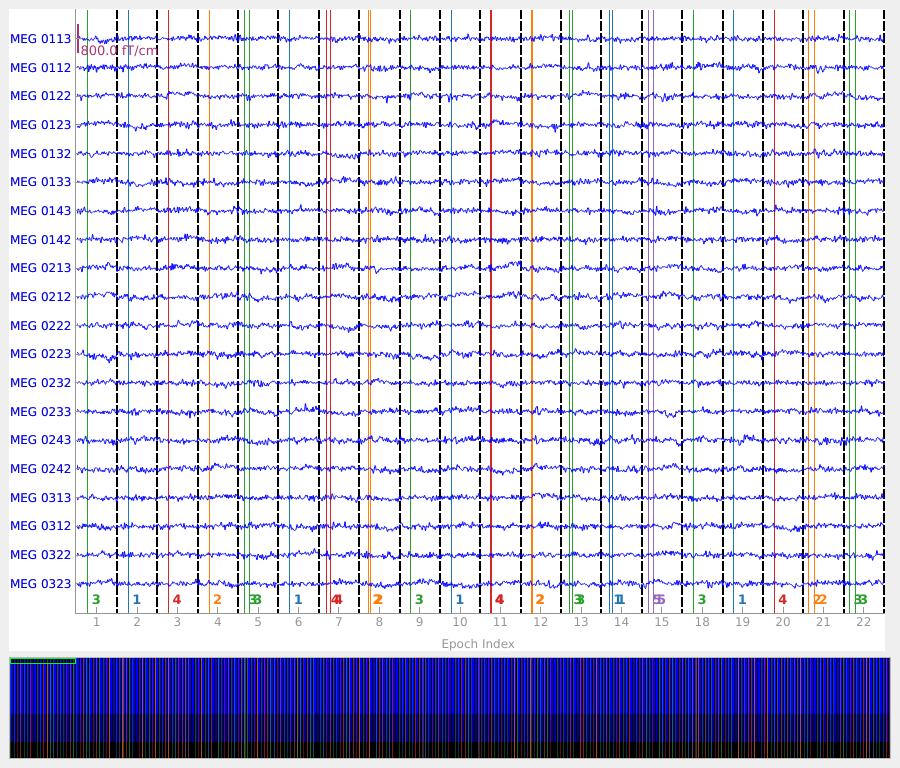```Using data from preloaded Raw for 319 events and 106 original time points ...
Rejecting  epoch based on EOG : ['EOG 061']
Rejecting  epoch based on EOG : ['EOG 061']
Rejecting  epoch based on EOG : ['EOG 061']
Rejecting  epoch based on EOG : ['EOG 061']
Rejecting  epoch based on EOG : ['EOG 061']
Rejecting  epoch based on EOG : ['EOG 061']
Rejecting  epoch based on EOG : ['EOG 061']
Rejecting  epoch based on EOG : ['EOG 061']
Rejecting  epoch based on EOG : ['EOG 061']
Rejecting  epoch based on EOG : ['EOG 061']
Rejecting  epoch based on EOG : ['EOG 061']
Rejecting  epoch based on EOG : ['EOG 061']
Rejecting  epoch based on EOG : ['EOG 061']
Rejecting  epoch based on EOG : ['EOG 061']
Rejecting  epoch based on EOG : ['EOG 061']
Rejecting  epoch based on EOG : ['EOG 061']
Rejecting  epoch based on MAG : ['MEG 1711']
Rejecting  epoch based on EOG : ['EOG 061']
Rejecting  epoch based on EOG : ['EOG 061']
Rejecting  epoch based on EOG : ['EOG 061']
Rejecting  epoch based on EOG : ['EOG 061']
Rejecting  epoch based on EOG : ['EOG 061']
Rejecting  epoch based on MAG : ['MEG 1711']
Rejecting  epoch based on EOG : ['EOG 061']
Rejecting  epoch based on EOG : ['EOG 061']
Rejecting  epoch based on EOG : ['EOG 061']
Rejecting  epoch based on EOG : ['EOG 061']
Rejecting  epoch based on EOG : ['EOG 061']
Rejecting  epoch based on EOG : ['EOG 061']
Rejecting  epoch based on EOG : ['EOG 061']
Rejecting  epoch based on EOG : ['EOG 061']
Rejecting  epoch based on EOG : ['EOG 061']
Rejecting  epoch based on EOG : ['EOG 061']
Rejecting  epoch based on EOG : ['EOG 061']
Rejecting  epoch based on EOG : ['EOG 061']
Rejecting  epoch based on EOG : ['EOG 061']
Rejecting  epoch based on EOG : ['EOG 061']
Rejecting  epoch based on EOG : ['EOG 061']
Rejecting  epoch based on EOG : ['EOG 061']
Rejecting  epoch based on EOG : ['EOG 061']
Rejecting  epoch based on EOG : ['EOG 061']
Rejecting  epoch based on EOG : ['EOG 061']
Rejecting  epoch based on EOG : ['EOG 061']
Rejecting  epoch based on EOG : ['EOG 061']
Rejecting  epoch based on EOG : ['EOG 061']
Rejecting  epoch based on EOG : ['EOG 061']
Rejecting  epoch based on EOG : ['EOG 061']
Rejecting  epoch based on EOG : ['EOG 061']
Rejecting  epoch based on EOG : ['EOG 061']
Rejecting  epoch based on EOG : ['EOG 061']
Rejecting  epoch based on EOG : ['EOG 061']
You seem to have overlapping epochs. Some event lines may be duplicated in the plot.
Using data from preloaded Raw for 20 events and 106 original time points ...
Using data from preloaded Raw for 20 events and 106 original time points ...
Using data from preloaded Raw for 20 events and 106 original time points ...
You seem to have overlapping epochs. Some event lines may be duplicated in the plot.
Computing rank from covariance with rank=None
Using tolerance 8.3e-14 (2.2e-16 eps * 59 dim * 6.3  max singular value)
Estimated rank (eeg): 58
EEG: rank 58 computed from 59 data channels with 1 projector
Computing rank from covariance with rank=None
Using tolerance 1.9e-13 (2.2e-16 eps * 203 dim * 4.3  max singular value)
GRAD: rank 203 computed from 203 data channels with 0 projectors
Computing rank from covariance with rank=None
Using tolerance 2.7e-14 (2.2e-16 eps * 102 dim * 1.2  max singular value)
Estimated rank (mag): 99
MAG: rank 99 computed from 102 data channels with 3 projectors
Using data from preloaded Raw for 20 events and 106 original time points ...
Using data from preloaded Raw for 20 events and 106 original time points ...
Using data from preloaded Raw for 20 events and 106 original time points ...
```

## Evoked data with whitening#

```evoked = epochs.average()
evoked.plot(time_unit="s")
evoked.plot(noise_cov=noise_cov, time_unit="s")
```
•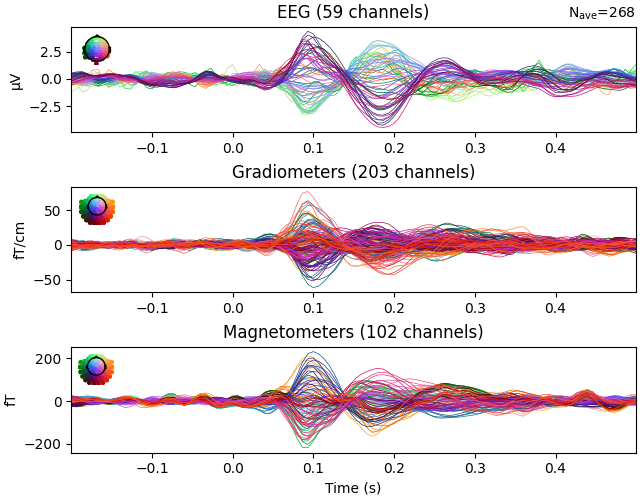•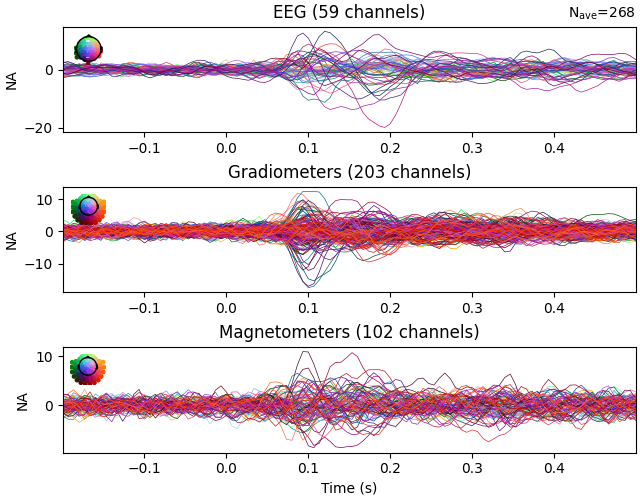```NOTE: pick_channels() is a legacy function. New code should use inst.pick(...).
Computing rank from covariance with rank=None
Using tolerance 8.3e-14 (2.2e-16 eps * 59 dim * 6.3  max singular value)
Estimated rank (eeg): 58
EEG: rank 58 computed from 59 data channels with 1 projector
Computing rank from covariance with rank=None
Using tolerance 1.9e-13 (2.2e-16 eps * 203 dim * 4.3  max singular value)
GRAD: rank 203 computed from 203 data channels with 0 projectors
Computing rank from covariance with rank=None
Using tolerance 2.7e-14 (2.2e-16 eps * 102 dim * 1.2  max singular value)
Estimated rank (mag): 99
MAG: rank 99 computed from 102 data channels with 3 projectors
```

## Evoked data with scaled whitening#

The `mne.Evoked.plot_white()` function takes an additional step of scaling the whitened plots to show how well the assumption of Gaussian noise is satisfied by the data:

```evoked.plot_white(noise_cov=noise_cov, time_unit="s")
```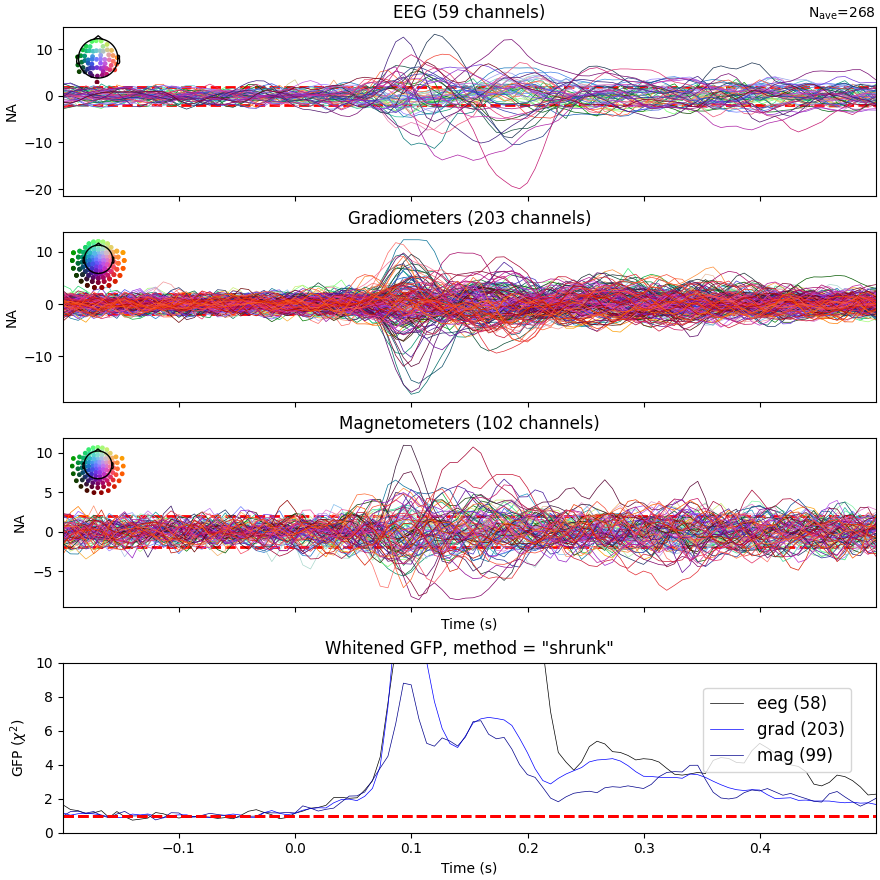```NOTE: pick_types() is a legacy function. New code should use inst.pick(...).
Computing rank from covariance with rank=None
Using tolerance 8.3e-14 (2.2e-16 eps * 59 dim * 6.3  max singular value)
Estimated rank (eeg): 58
EEG: rank 58 computed from 59 data channels with 1 projector
Computing rank from covariance with rank=None
Using tolerance 1.9e-13 (2.2e-16 eps * 203 dim * 4.3  max singular value)
GRAD: rank 203 computed from 203 data channels with 0 projectors
Computing rank from covariance with rank=None
Using tolerance 2.7e-14 (2.2e-16 eps * 102 dim * 1.2  max singular value)
Estimated rank (mag): 99
MAG: rank 99 computed from 102 data channels with 3 projectors
Created an SSP operator (subspace dimension = 4)
Computing rank from covariance with rank={'eeg': 58, 'grad': 203, 'mag': 99, 'meg': 302}
Setting small MEG eigenvalues to zero (without PCA)
Setting small EEG eigenvalues to zero (without PCA)
Created the whitener using a noise covariance matrix with rank 360 (4 small eigenvalues omitted)
```

## Topographic plot with whitening#

```evoked.comment = "All trials"
evoked.plot_topo(title="Evoked data")
evoked.plot_topo(noise_cov=noise_cov, title="Whitened evoked data")
```
•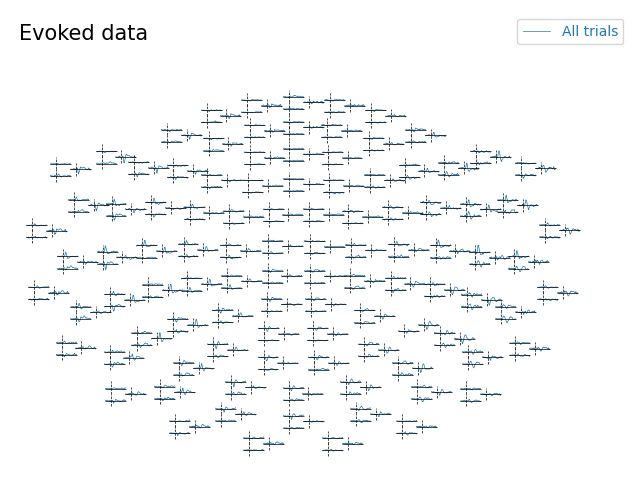•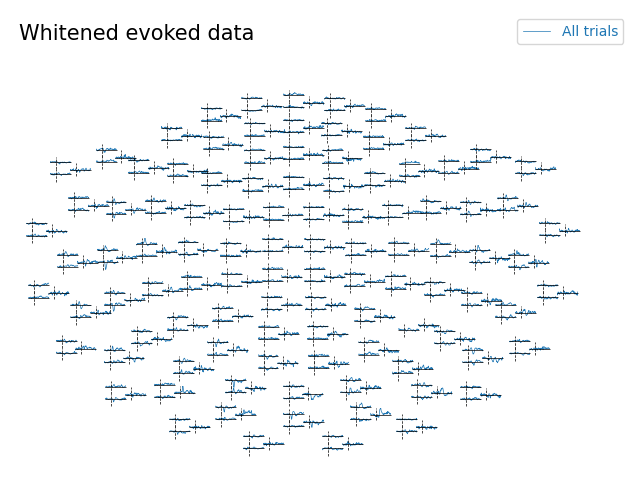```    Created an SSP operator (subspace dimension = 4)
Computing rank from covariance with rank=None
Using tolerance 3.6e-13 (2.2e-16 eps * 305 dim * 5.3  max singular value)
Estimated rank (mag + grad): 302
MEG: rank 302 computed from 305 data channels with 3 projectors
Using tolerance 8.3e-14 (2.2e-16 eps * 59 dim * 6.3  max singular value)
Estimated rank (eeg): 58
EEG: rank 58 computed from 59 data channels with 1 projector
Setting small MEG eigenvalues to zero (without PCA)
Setting small EEG eigenvalues to zero (without PCA)
Created the whitener using a noise covariance matrix with rank 360 (4 small eigenvalues omitted)
```

Total running time of the script: (0 minutes 19.768 seconds)

Estimated memory usage: 169 MB

Gallery generated by Sphinx-Gallery